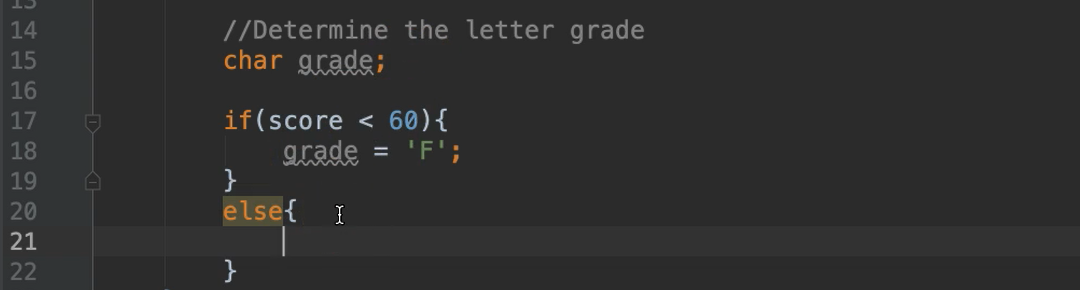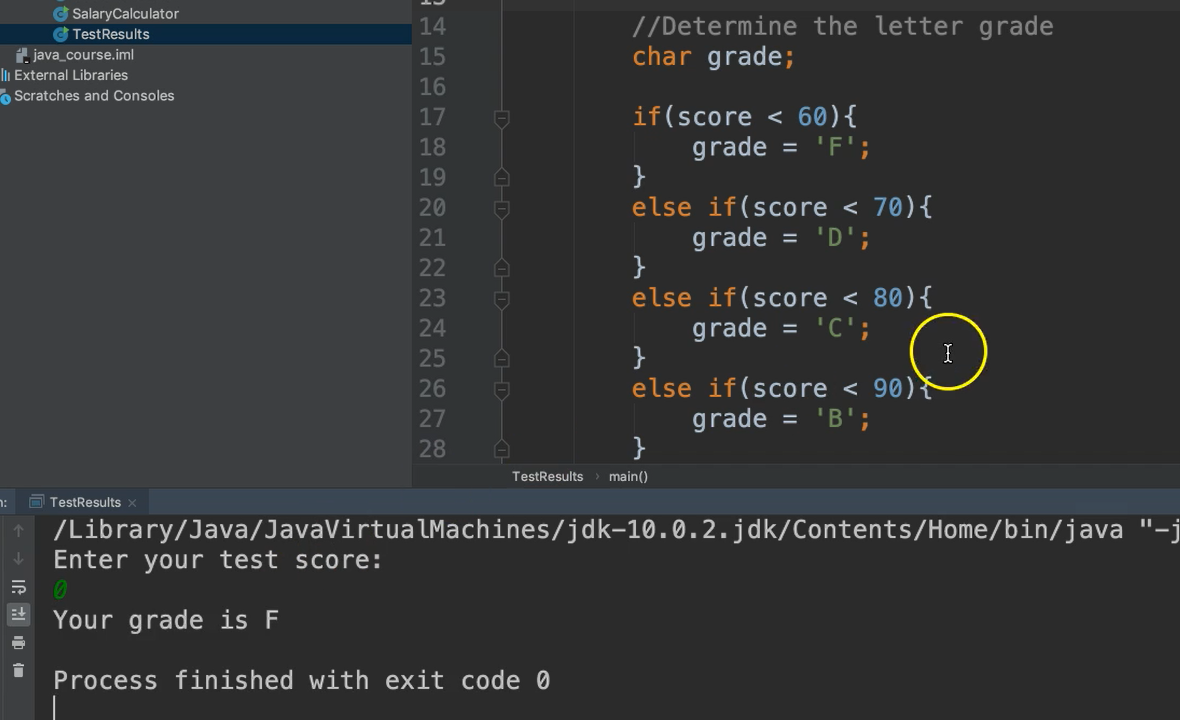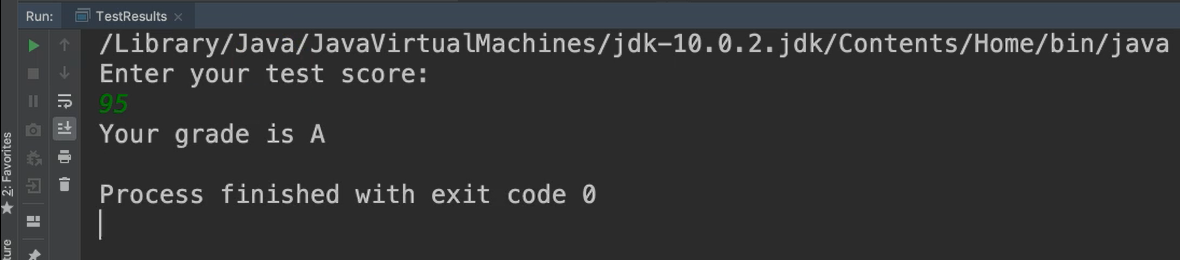Transcripted Summary

The if-else-if statement is used when there are more than two possible paths.

The If-Else-If _Statement

If situation A occurs, do something.

Else if situation B occurs, do something else.

Else if situation C occurs, do something else.

For example, if situation A occurs, let's go down one path; else, if situation B occurs, let's go down another path; else, if situation C occurs, let's go down a different path.

Let's look at an example.Given a numeric test score, we need to figure out the letter grade for that test score.

Let's code it.

We’ll create a new class called `TestResults` in the `chapter3` package. Inside of here, let’s go ahead and make our main method. For our design, we want to get the test score from the user and then determine the letter grade.

``````package chapter3;

/*
* IF-ELSE-IF
* Display the letter grade for a student based on their test score.
*/
public class TestResults {

public static void main(String args[]){

//Get the test score

}
}
``````

We don’t know what the test score is yet, so, we'll ask the user.

``````//Get the test score
``````

And we will input the data. We're asking them for a numeric score and it could be a decimal, so let’s store this in a variable of type `double`. Don’t forget to import Scanner.

``````Scanner scanner = new Scanner(System.in);
double score = scanner.nextDouble();
scanner.close();
``````

And now we want to determine the grade.

We have 5 grades we can choose from: A, B, C, D, or F. So, that's 5 different possible paths. So far, we've looked at if, and_ if-else_, so it really only covers 2 paths at max. What do we do when we have something like 5 different paths?

Let's take a look.

We're going to introduce a new data type called `char`, and this stands for character.

Character Data Type

The `char` data type holds exactly one character. So, it's similar to a String, meaning it holds some type of text. However, it's only one character of text. `char` data types use the single quotation marks; double quotation marks are for String data type, and single is for `char`.

We'll call this `grade`.

``````//Determine the letter grade
``````

I'm going to declare this variable, but I don't have a value yet. I don't know what the value is until we go through our decision structure. So, it’s totally legal to declare the variable without initializing its value. I know that I need this variable, and once I figure out what the value is, then I'll assign it at that point.

Now with our decision structure.

Let's say if the score is less than 60, then we're going to update that grade variable and assign it the letter F.

``````if(score < 60){
}
``````

That covers the case of less than 60, but if it’s anything above 60, we don’t have a value for `grade` yet. So, let’s make additional branches.

Before, we looked at _if/else _branches, like this:And I told you that the specified condition (`score < 60`) is for both the if and the else paths; meaning if the condition is true, the if branch is executed, and if it is false, the else branch is executed.

But, when we're looking at multiple paths, more than 2, we possibly need to add a new condition, and that's what we need to do in this case. As opposed to just saying `else`, we're going to say `else if`, and include yet another condition.

We can say if the score is less than 70, then the grade equals D.

``````if(score < 60){
}
else if(score < 70){
}
``````

We have two conditions, so it's going to look at the very first one. If that is false, it's going to go to the next one. But it doesn't go inside by default, it has to evaluate this second condition and determine if it's true or false.

Okay, let's keep going.

We're going to have another `else if`, this time with the condition of the score being less than 80. If so, grade will equal to C.

``````else if(score < 80){
}
``````

Another one, `else if``, score is less than 90, grade is equal to B.

``````else if(score < 90){
}
``````

Now finally, if it's anything other than this, we've covered from 90 on down, if it's anything other than that, it's going to be an A.

So, I don't need a condition here; I can simply add the last `else`. That way, all my bases are covered.

``````else{
}
``````

If I were to add a condition on the last one, it would be okay. However, if the `score` happens to be anything that I didn’t think of as the programmer, then none of these conditions would've been met.

So now I have all of my bases covered because if none of these conditions were met, then at least I have a fallback (the `else` without a condition).

We've assigned the grade based on the score. Now let's just print it out.

``````System.out.println("Your grade is " + grade);
``````

And notice we can also append this char data type to this String.

Let's give it a go.

### # TestResults.java

``````package chapter3;

import java.util.Scanner;

/*
* IF-ELSE-IF
* Display the letter grade for a student based on their test score.
*/
public class TestResults {

public static void main(String args[]){

//Get the test score
Scanner scanner = new Scanner(System.in);
double score = scanner.nextDouble();
scanner.close();

if(score < 60){
}
else if(score < 70){
}
else if(score < 80){
}
else if(score < 90){
}
else{
}

}
}
``````

So, let's say we have a test score of 0. We expect an F.Perfect.

Let's do it again, this time let's say maybe 55. Also, an F.

Let's try for a 95. That's an A.And let's just try one more, say maybe like a 75. We get a C.

So that is the if-else-if.